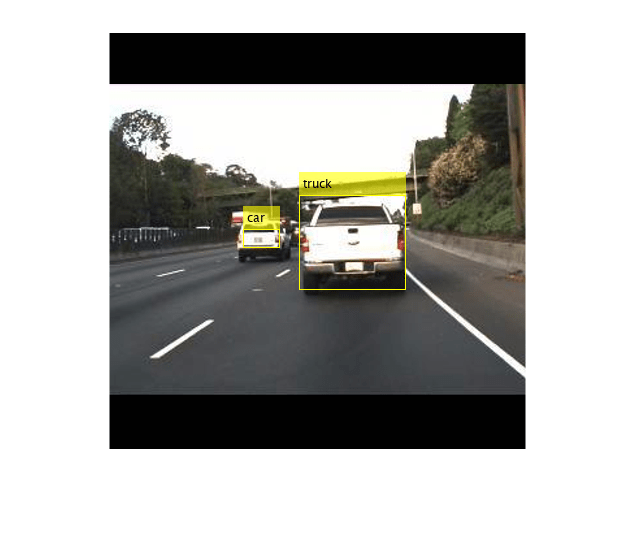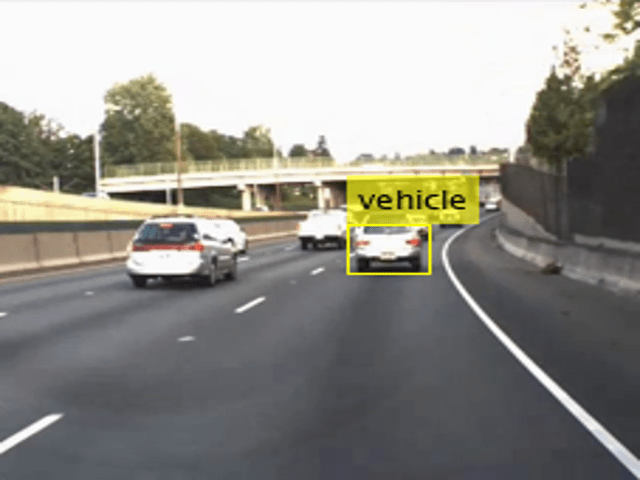Main Content

# Code Generation For Object Detection Using YOLO v3 Deep Learning

This example shows how to generate CUDA® MEX for a you only look once (YOLO) v3 object detector with custom layers. YOLO v3 improves upon YOLO v2 by adding detection at multiple scales to help detect smaller objects. Moreover, the loss function used for training is separated into mean squared error for bounding box regression and binary cross-entropy for object classification to help improve detection accuracy. This example uses the YOLO v3 network trained in the Object Detection Using YOLO v3 Deep Learning example from the Computer Vision Toolbox (TM). For more information, see Object Detection Using YOLO v3 Deep Learning (Computer Vision Toolbox).

### Third-Party Prerequisites

#### Required

• CUDA enabled NVIDIA® GPU and compatible driver.

#### Optional

For non-MEX builds such as static, dynamic libraries or executables, this example has the following additional requirements.

### Verify GPU Environment

To verify that the compilers and libraries for running this example are set up correctly, use the `coder.checkGpuInstall` function.

```envCfg = coder.gpuEnvConfig('host'); envCfg.DeepLibTarget = 'cudnn'; envCfg.DeepCodegen = 1; envCfg.Quiet = 1; coder.checkGpuInstall(envCfg);```

### YOLO v3 Network

The YOLO v3 network in this example is based on `squeezenet` (Deep Learning Toolbox), and uses the feature extraction network in SqueezeNet with the addition of two detection heads at the end. The second detection head is twice the size of the first detection head, so it is better able to detect small objects. Note that any number of detection heads of different sizes can be specified based on the size of the objects to be detected. The YOLO v3 network uses anchor boxes estimated using training data to have better initial priors corresponding to the type of data set and to help the network learn to predict the boxes accurately. For information about anchor boxes, see Anchor Boxes for Object Detection (Computer Vision Toolbox).

The YOLO v3 network in this example is illustrated in the following diagram.Each detection head predicts the bounding box coordinates (x, y, width, height), object confidence, and class probabilities for the respective anchor box masks. Therefore, for each detection head, the number of output filters in the last convolution layer is the number of anchor box mask times the number of prediction elements per anchor box. The detection heads comprise the output layer of the network.

### Pretrained YOLO v3 Network

The YOLO v3 network used in this example was trained using the steps described in Object Detection Using YOLO v3 Deep Learning (Computer Vision Toolbox).

```matFile = 'yolov3SqueezeNetVehicleExample.mat'; pretrained = load(matFile); net = pretrained.net;```

YOLO v3 network uses a `resize2dLayer` (Image Processing Toolbox) to resize the 2-D input image by replicating the neighboring pixel values by a scaling factor of 2. The resize2DLayer is implemented as a custom layer supported for code generation. For more information, see Define Custom Deep Learning Layer for Code Generation (Deep Learning Toolbox).

### The `yolov3Detect` Entry-Point Function

The `yolov3Detect` entry-point function takes an input image and passes it to a trained network for prediction through the `yolov3Predict` function. The `yolov3Predict` function loads the network object from the MAT-file into a persistent variable and reuses the persistent object for subsequent prediction calls. Specifically, the function uses the `dlnetwork` (Deep Learning Toolbox) representation of the network trained in the Object Detection Using YOLO v3 Deep Learning (Computer Vision Toolbox) example. The predictions from the YOLO v3 grid cell coordinates obtained from the `yolov3Predict` calls are then converted to bounding box coordinates by using the supporting functions `generateTiledAnchors` and `applyAnchorBoxOffsets`.

`type('yolov3Detect.m')`
```function [bboxes,scores,labelsIndex] = yolov3Detect(matFile, im,... networkInputSize, networkOutputs, confidenceThreshold,... overlapThreshold, classes) % The yolov3Detect function detects the bounding boxes, scores, and % labelsIndex in an image. %#codegen %% Preprocess Data % This example applies all the preprocessing transforms to the data set % applied during training, except data augmentation. Because the example % uses a pretrained YOLO v3 network, the input data must be representative % of the original data and left unmodified for unbiased evaluation. % Specifically the following preprocessing operations are applied to the % input data. % 1. Resize the images to the network input size, as the images are % bigger than networkInputSize. 2. Scale the image pixels in the range % [0 1]. 3. Convert the resized and rescaled image to a dlarray object. im = dlarray(preprocessData(im, networkInputSize), "SSCB"); imageSize = size(im,[1,2]); %% Define Anchor Boxes % Specify the anchor boxes estimated on the basis of the preprocessed % training data used when training the YOLO v3 network. These anchor box % values are same as mentioned in "Object Detection Using YOLO v3 Deep % Learning" example. For details on estimating anchor boxes, see "Anchor % Boxes for Object Detection". anchors = [ 41 34; 163 130; 98 93; 144 125; 33 24; 69 66]; % Specify anchorBoxMasks to select anchor boxes to use in both the % detection heads of the YOLO v3 network. anchorBoxMasks is a cell array of % size M-by-1, where M denotes the number of detection heads. Each % detection head consists of a 1-by-N array of row index of anchors in % anchorBoxes, where N is the number of anchor boxes to use. Select anchor % boxes for each detection head based on size-use larger anchor boxes at % lower scale and smaller anchor boxes at higher scale. To do so, sort the % anchor boxes with the larger anchor boxes first and assign the first % three to the first detection head and the next three to the second % detection head. area = anchors(:, 1).*anchors(:, 2); [~, idx] = sort(area, 'descend'); anchors = anchors(idx, :); anchorBoxMasks = {[1,2,3] [4,5,6] }; %% Predict on Yolov3 % Predict and filter the detections based on confidence threshold. predictions = yolov3Predict(matFile,im,networkOutputs,anchorBoxMasks); %% Generate Detections % indices corresponding to x,y,w,h predictions for bounding boxes anchorIndex = 2:5; tiledAnchors = generateTiledAnchors(predictions,anchors,anchorBoxMasks,... anchorIndex); predictions = applyAnchorBoxOffsets(tiledAnchors, predictions,... networkInputSize, anchorIndex); [bboxes,scores,labelsIndex] = generateYOLOv3DetectionsForCodegen(predictions,... confidenceThreshold, overlapThreshold, imageSize, classes); end function YPredCell = yolov3Predict(matFile,im,networkOutputs,anchorBoxMask) % Predict the output of network and extract the confidence, x, y, % width, height, and class. % load the deep learning network for prediction persistent net; if isempty(net) net = coder.loadDeepLearningNetwork(matFile); end YPredictions = cell(coder.const(networkOutputs), 1); [YPredictions{:}] = predict(net, im); YPredCell = extractPredictions(YPredictions, anchorBoxMask); % Apply activation to the predicted cell array. YPredCell = applyActivations(YPredCell); end ```

### Evaluate the Entry-Point Function for Object Detection

Follow these steps to evaluate the entry-point function on an image from the test data.

• Specify the confidence threshold as 0.5 to keep only detections with confidence scores above this value.

• Specify the overlap threshold as 0.5 to remove overlapping detections.

• Read an image from the input data.

• Use the entry-point function `yolov3Detect` to get the predicted bounding boxes, confidence scores, and class labels.

• Display the image with bounding boxes and confidence scores.

Define the desired thresholds.

```confidenceThreshold = 0.5; overlapThreshold = 0.5;```

Specify the network input size of the trained network and the number of network outputs.

```networkInputSize = [227 227 3]; networkOutputs = numel(net.OutputNames);```

Read the example image data obtained from the labeled data set from the Object Detection Using YOLO v3 Deep Learning (Computer Vision Toolbox) example. This image contains one instance of an object of type vehicle.

`I = imread('vehicleImage.jpg');`

Specify the class names.

`classNames = {'vehicle'};`

Invoke the detect method on YOLO v3 network and display the results.

```[bboxes,scores,labelsIndex] = yolov3Detect(matFile,I,... networkInputSize,networkOutputs,confidenceThreshold,overlapThreshold,classNames); labels = classNames{labelsIndex}; % Display the detections on the image IAnnotated = insertObjectAnnotation(I,'rectangle',bboxes,[labels ' - ' num2str(scores)]); figure imshow(IAnnotated)```### Generate CUDA MEX

To generate CUDA® code for the `yolov3Detect` entry-point function, create a GPU code configuration object for a MEX target and set the target language to C++. Use the `coder.DeepLearningConfig` function to create a CuDNN deep learning configuration object and assign it to the `DeepLearningConfig` property of the GPU code configuration object.

```cfg = coder.gpuConfig('mex'); cfg.TargetLang = 'C++'; cfg.DeepLearningConfig = coder.DeepLearningConfig(TargetLibrary='cudnn'); args = {coder.Constant(matFile),I,coder.Constant(networkInputSize),... coder.Constant(networkOutputs),confidenceThreshold,... overlapThreshold,classNames}; codegen -config cfg yolov3Detect -args args -report```
```Code generation successful: View report ```

To generate CUDA® code for TensorRT target create and use a TensorRT deep learning configuration object instead of the CuDNN configuration object. Similarly, to generate code for MKLDNN target, create a CPU code configuration object and use MKLDNN deep learning configuration object as its `DeepLearningConfig` property.

### Run the Generated MEX

Call the generated CUDA MEX with the same image input `I `as before and display the results.

```[bboxes,scores,labelsIndex] = yolov3Detect_mex(matFile,I,... networkInputSize,networkOutputs,confidenceThreshold,... overlapThreshold,classNames); labels = classNames{labelsIndex}; figure; IAnnotated = insertObjectAnnotation(I,'rectangle',bboxes,[labels ' - ' num2str(scores)]); imshow(IAnnotated);```### Utility Functions

The utillity functions listed below are based on the ones used in Object Detection Using YOLO v3 Deep Learning (Computer Vision Toolbox) example and modified to make the utility functions suitable for code generation.

`type('applyActivations.m')`
```function YPredCell = applyActivations(YPredCell) %#codegen numCells = size(YPredCell, 1); for iCell = 1:numCells for idx = 1:3 YPredCell{iCell, idx} = sigmoidActivation(YPredCell{iCell,idx}); end end for iCell = 1:numCells for idx = 4:5 YPredCell{iCell, idx} = exp(YPredCell{iCell, idx}); end end for iCell = 1:numCells YPredCell{iCell, 6} = sigmoidActivation(YPredCell{iCell, 6}); end end function out = sigmoidActivation(x) out = 1./(1+exp(-x)); end ```
`type('extractPredictions.m')`
```function predictions = extractPredictions(YPredictions, anchorBoxMask) %#codegen numPredictionHeads = size(YPredictions, 1); predictions = cell(numPredictionHeads,6); for ii = 1:numPredictionHeads % Get the required info on feature size. numChannelsPred = size(YPredictions{ii},3); numAnchors = size(anchorBoxMask{ii},2); numPredElemsPerAnchors = numChannelsPred/numAnchors; allIds = (1:numChannelsPred); stride = numPredElemsPerAnchors; endIdx = numChannelsPred; YPredictionsData = extractdata(YPredictions{ii}); % X positions. startIdx = 1; predictions{ii,2} = YPredictionsData(:,:,startIdx:stride:endIdx,:); xIds = startIdx:stride:endIdx; % Y positions. startIdx = 2; predictions{ii,3} = YPredictionsData(:,:,startIdx:stride:endIdx,:); yIds = startIdx:stride:endIdx; % Width. startIdx = 3; predictions{ii,4} = YPredictionsData(:,:,startIdx:stride:endIdx,:); wIds = startIdx:stride:endIdx; % Height. startIdx = 4; predictions{ii,5} = YPredictionsData(:,:,startIdx:stride:endIdx,:); hIds = startIdx:stride:endIdx; % Confidence scores. startIdx = 5; predictions{ii,1} = YPredictionsData(:,:,startIdx:stride:endIdx,:); confIds = startIdx:stride:endIdx; % Accummulate all the non-class indexes nonClassIds = [xIds yIds wIds hIds confIds]; % Class probabilities. % Get the indexes which do not belong to the nonClassIds classIdx = setdiff(allIds, nonClassIds, 'stable'); predictions{ii,6} = YPredictionsData(:,:,classIdx,:); end end ```
`type('generateTiledAnchors.m')`
```function tiledAnchors = generateTiledAnchors(YPredCell,anchorBoxes,... anchorBoxMask,anchorIndex) % Generate tiled anchor offset for converting the predictions from the YOLO % v3 grid cell coordinates to bounding box coordinates %#codegen numPredictionHeads = size(YPredCell,1); tiledAnchors = cell(numPredictionHeads, size(anchorIndex, 2)); for i=1:numPredictionHeads anchors = anchorBoxes(anchorBoxMask{i}, :); [h,w,~,n] = size(YPredCell{i,1}); [tiledAnchors{i,2},tiledAnchors{i,1}] = ndgrid(0:h-1,0:w-1,... 1:size(anchors,1),1:n); [~,~,tiledAnchors{i,3}] = ndgrid(0:h-1,0:w-1,anchors(:,2),1:n); [~,~,tiledAnchors{i,4}] = ndgrid(0:h-1,0:w-1,anchors(:,1),1:n); end end ```
`type('applyAnchorBoxOffsets.m')`
```function YPredCell = applyAnchorBoxOffsets(tiledAnchors,YPredCell,... inputImageSize,anchorIndex) % Convert the predictions from the YOLO v3 grid cell coordinates to % bounding box coordinates %#codegen for i=1:size(YPredCell,1) [h,w,~,~] = size(YPredCell{i,1}); YPredCell{i,anchorIndex(1)} = (tiledAnchors{i,1}+... YPredCell{i,anchorIndex(1)})./w; YPredCell{i,anchorIndex(2)} = (tiledAnchors{i,2}+... YPredCell{i,anchorIndex(2)})./h; YPredCell{i,anchorIndex(3)} = (tiledAnchors{i,3}.*... YPredCell{i,anchorIndex(3)})./inputImageSize(2); YPredCell{i,anchorIndex(4)} = (tiledAnchors{i,4}.*... YPredCell{i,anchorIndex(4)})./inputImageSize(1); end end ```
`type('preprocessData.m')`
```function image = preprocessData(image,targetSize) % Resize the images and scale the pixels to between 0 and 1. %#codegen imgSize = size(image); % Convert an input image with single channel to 3 channels. if numel(imgSize) < 1 image = repmat(image,1,1,3); end image = im2single(imresize(image,coder.const(targetSize(1:2)))); end ```

### References

1. Redmon, Joseph, and Ali Farhadi. “YOLOv3: An Incremental Improvement.” Preprint, submitted April 8, 2018. https://arxiv.org/abs/1804.02767.

## Support

#### Generating CUDA Code from MATLAB: Accelerating Embedded Vision and Deep Learning Algorithms on GPUs

Download white paper